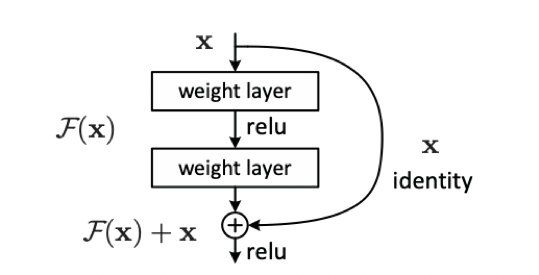# ResNet¶

## 1. 更深层次的网络？¶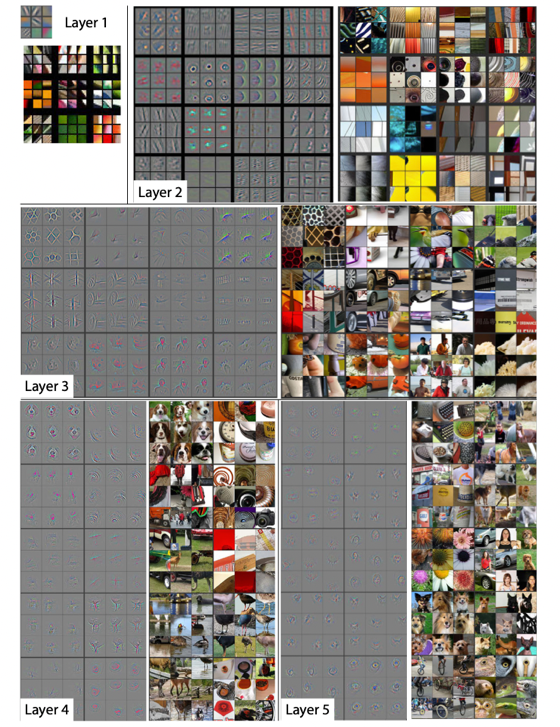## 2. 为什么深度网络不仅仅是层数的堆叠？¶

### 2.1 梯度消失 or 爆炸¶

$\begin{split} z_{i+1} = w_ia_i+b_i\\ a_{i+1} = \sigma(z_{i+1}) \end{split}$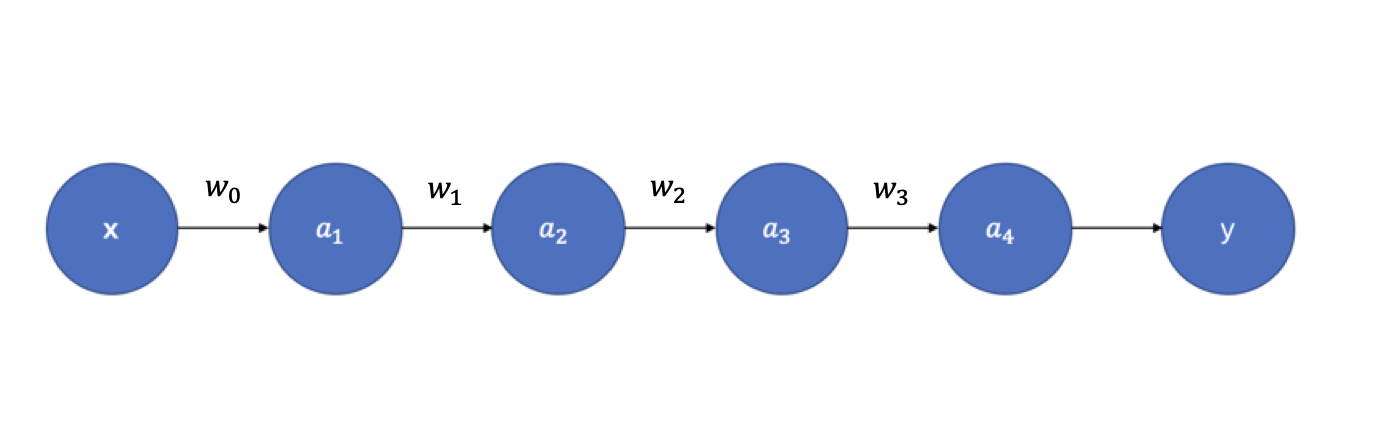$\begin{split} \frac{\partial y}{\partial a_1} = \frac{\partial y}{\partial a_4}\frac{\partial a_4}{\partial z_4}\frac{\partial z_4}{\partial a_3}\frac{\partial a_3}{\partial z_3}\frac{\partial z_3}{\partial a_2}\frac{\partial a_2}{\partial z_2}\frac{\partial z_2}{\partial a_1} \\ = \frac{\partial y}{\partial a_4}\sigma^{'}(z_4)w_3\sigma^{'}(z_3)w_2\sigma^{'}(z_2)w_1 \end{split}$

Sigmoid 函数的导数 $$\sigma^{'}(x)$$图3 所示：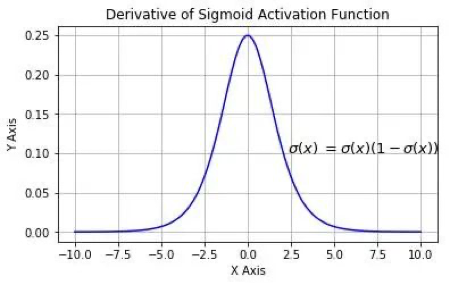### 2.2 网络退化¶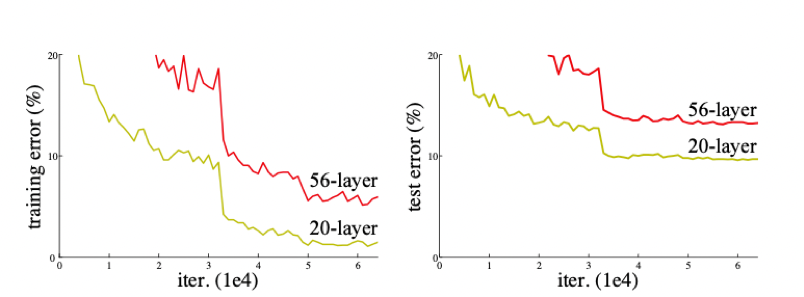## 3. 残差块¶